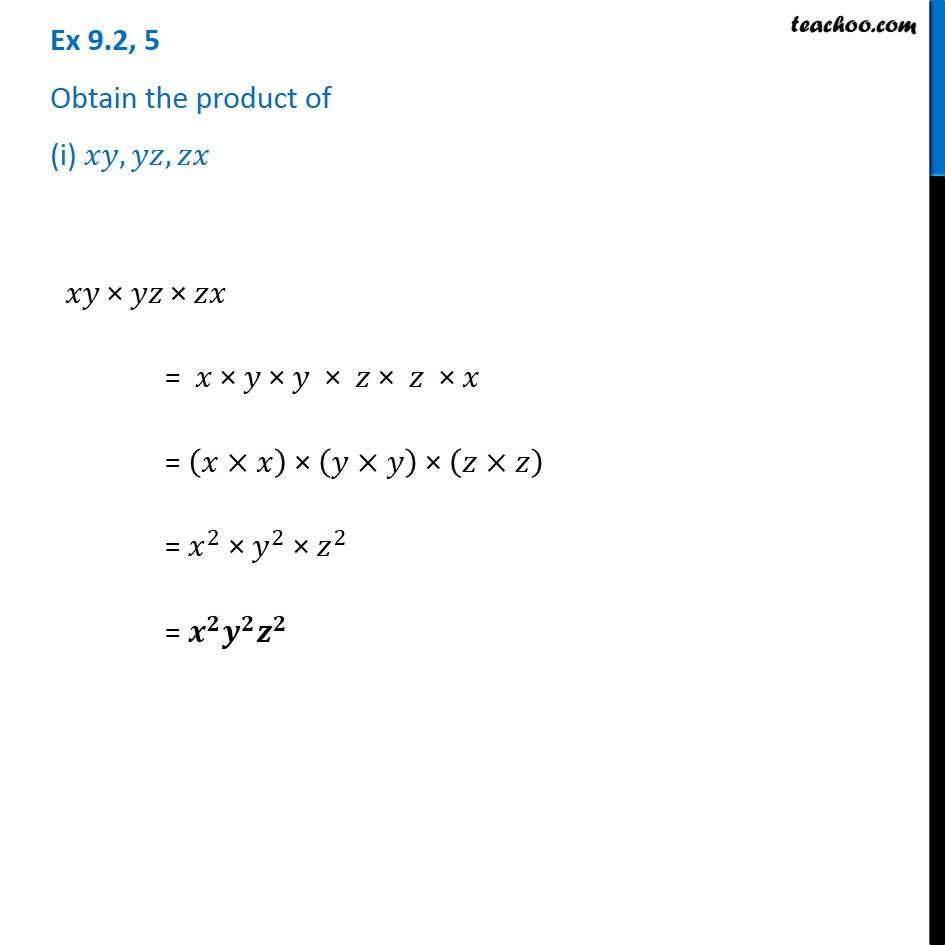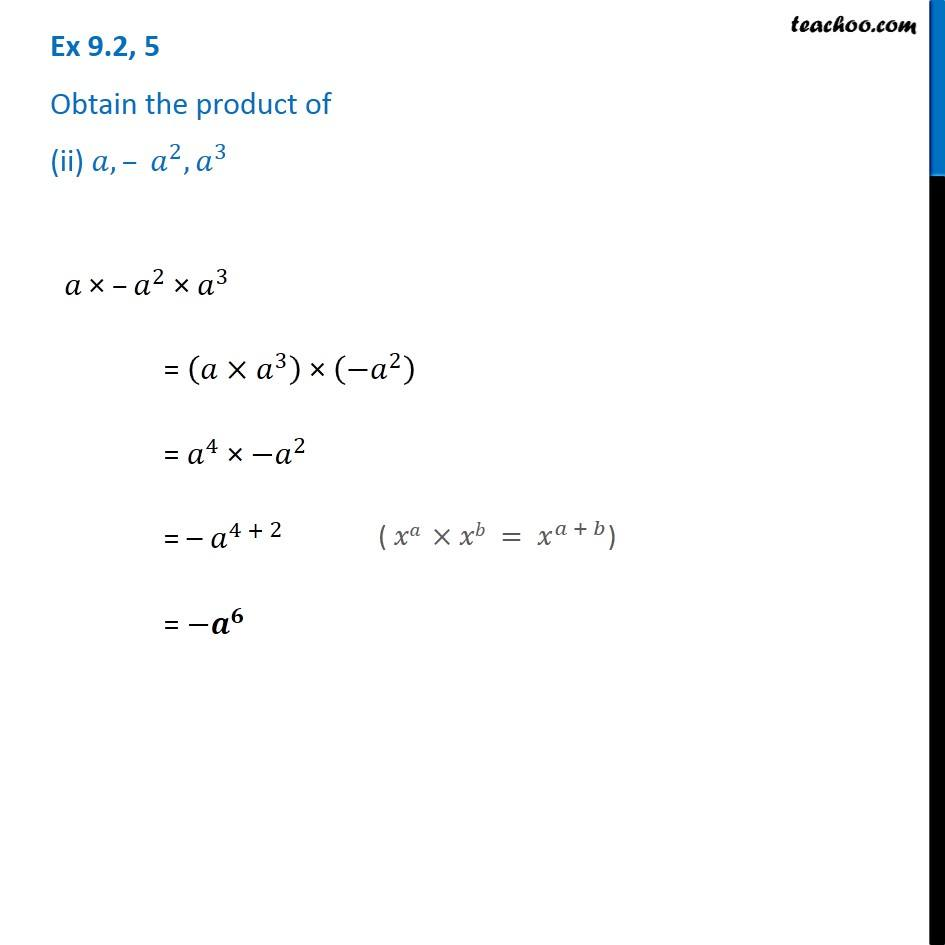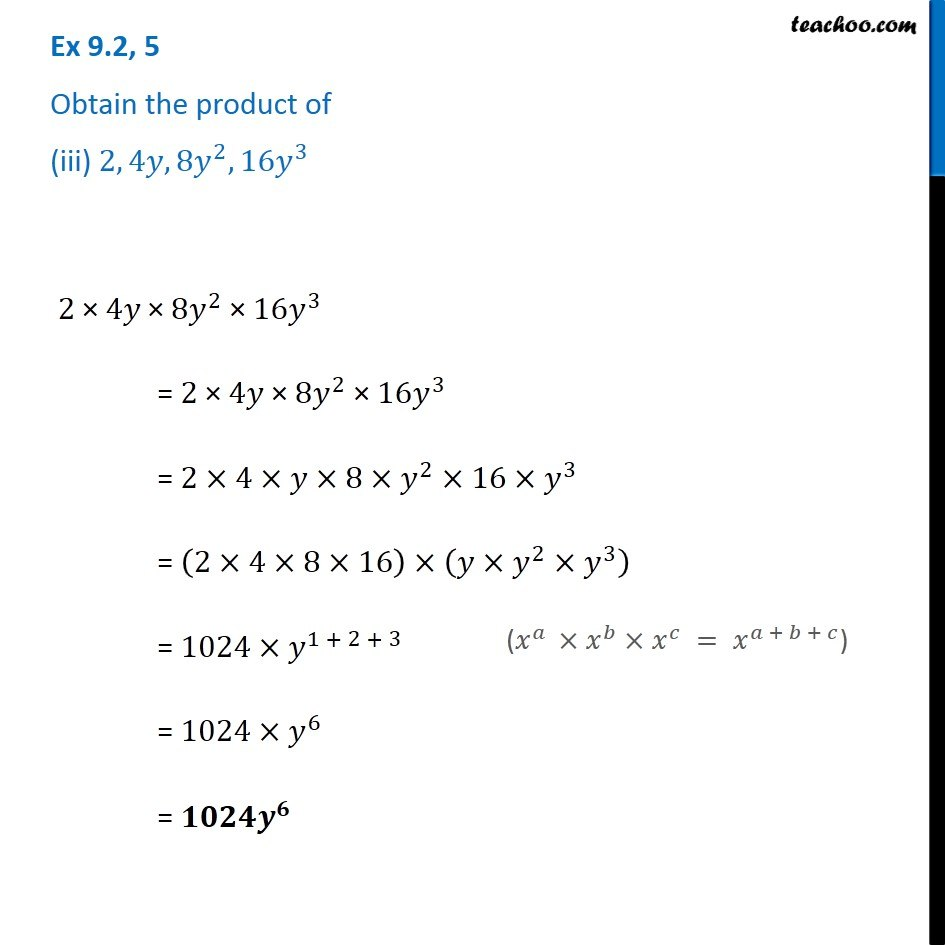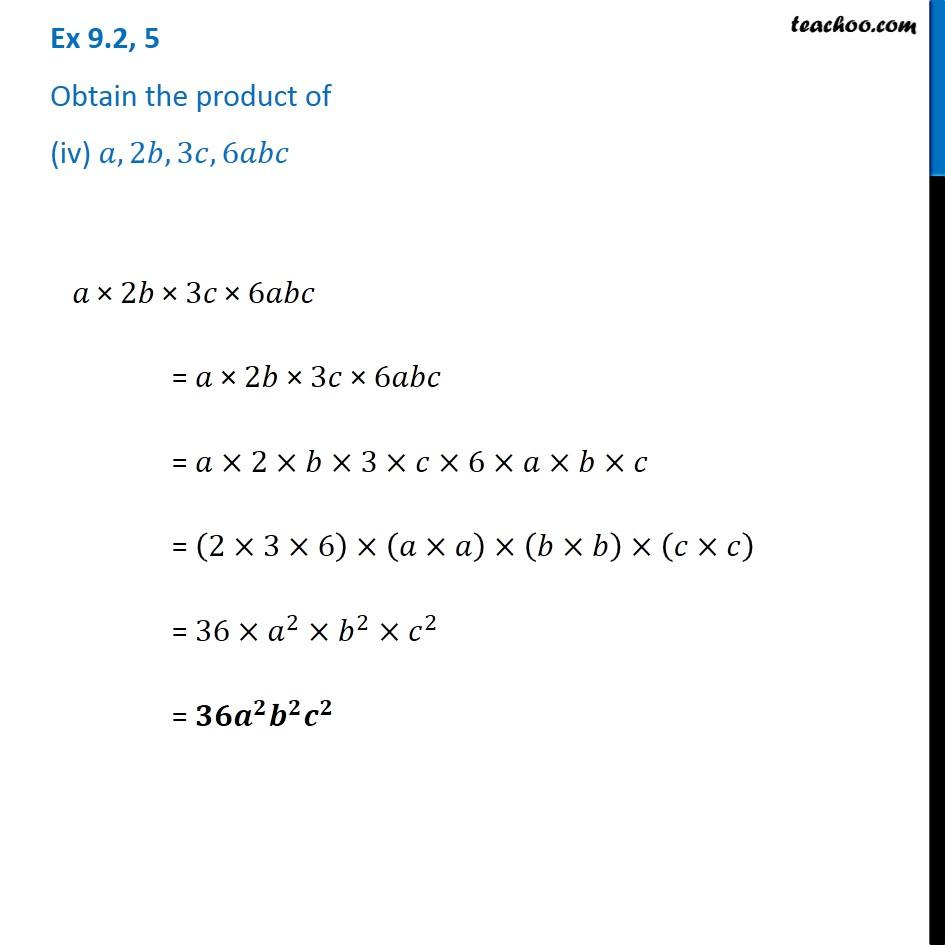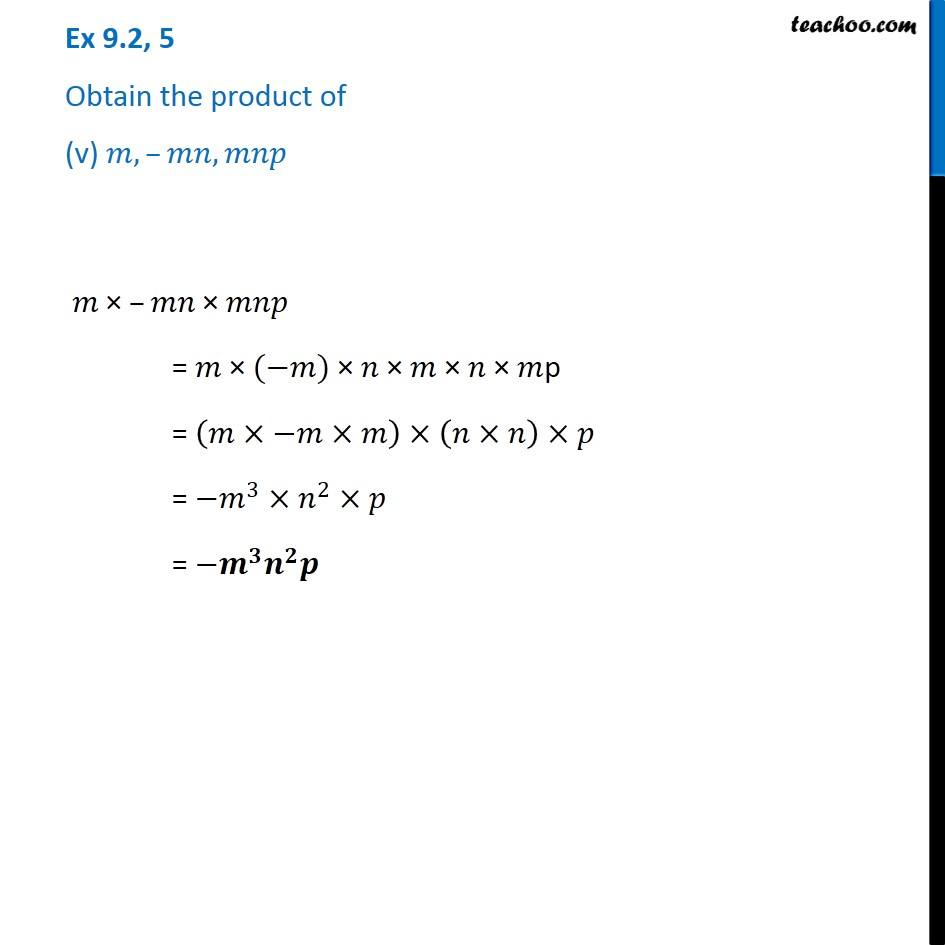Subscribe to our Youtube Channel - https://you.tube/teachoo

1. Chapter 9 Class 8 Algebraic Expressions and Identities
2. Serial order wise
3. Ex 9.2

Transcript

Ex 9.2, 5 Obtain the product of (i) 𝑥𝑦, 𝑦𝑧, 𝑧𝑥 𝑥𝑦" × " 𝑦𝑧" ×" 𝑧𝑥 = 𝑥 × 𝑦 × 𝑦 × 𝑧 × 𝑧 × 𝑥 = (𝑥×𝑥) × (𝑦×𝑦) × (𝑧×𝑧) = 𝑥^2 × 𝑦^2 × 𝑧^2 = 𝒙^𝟐 𝒚^𝟐 𝒛^𝟐 Ex 9.2, 5 Obtain the product of (ii) 𝑎, – 𝑎^2, 𝑎^3 𝑎" ×" –𝑎^2 "× " 𝑎^3 = (𝑎×𝑎^3 ) × (−𝑎^2 ) = 𝑎^4 × 〖−𝑎〗^2 = – 𝑎^(4 + 2) = −𝒂^𝟔 ( 𝑥𝑎 ×𝑥𝑏 = 𝑥^(𝑎 + 𝑏)) Ex 9.2, 5 Obtain the product of (iii) 2, 4𝑦, 8𝑦^2, 16𝑦^3 2" ×" 4𝑦" ×" 8𝑦^2 " ×" 16𝑦^3 = 2 × 4𝑦 × 8𝑦^2 × 16𝑦^3 = 2×4×𝑦×8×𝑦^2×16×𝑦^3 = (2×4×8×16)×(𝑦×𝑦^2×𝑦^3 ) = 1024×𝑦^(1 + 2 + 3) = 1024×𝑦^6 = 𝟏𝟎𝟐𝟒𝒚^𝟔 (𝑥^𝑎 ×𝑥^𝑏×𝑥^𝑐 = 𝑥^(𝑎 + 𝑏 + 𝑐)) Ex 9.2, 5 Obtain the product of (iv) 𝑎, 2𝑏, 3𝑐, 6𝑎𝑏𝑐 𝑎" ×" 2𝑏" × " 3𝑐" × " 6𝑎𝑏𝑐 = 𝑎 × 2𝑏 × 3𝑐 × 6𝑎𝑏𝑐 = 𝑎×2×𝑏×3×𝑐×6×𝑎×𝑏×𝑐 = (2×3×6)×(𝑎×𝑎)×(𝑏×𝑏)×(𝑐×𝑐) = 36×𝑎^2×𝑏^2×𝑐^2 = 𝟑𝟔𝒂^𝟐 𝒃^𝟐 𝒄^𝟐 Ex 9.2, 5 Obtain the product of (v) 𝑚, –𝑚𝑛, 𝑚𝑛𝑝 𝑚" × "–𝑚𝑛" ×" 𝑚𝑛𝑝 = 𝑚 × (−𝑚) × 𝑛 × 𝑚 × 𝑛 × 𝑚p = (𝑚×−𝑚×𝑚)×(𝑛×𝑛)×𝑝 = −𝑚^3×𝑛^2×𝑝 = −𝒎^𝟑 𝒏^𝟐 𝒑

Ex 9.2